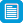# Search results

## Refine by topic

Main topic Specific topic
Related topic
Listed under:  Mathematics  >  Geometry  >  Shapes (Geometry)  >  Triangles (Shapes)### reSolve: Mechanical Linkages: Geometry Proofs

This series of six lessons explores geometry proofs using real world contexts focused on the dynamics of linkages and moving joints. Students use physical models and computer simulations, the lessons move from a view of geometry as a study to static diagrams to encompass movement. Each lesson is outlined in detail including ...### reSolve: Mechanical Linkages: Similar Triangles

This sequence of lessons explores the geometry of similar triangles using two real world objects: ironing boards and pantographs. In the first lesson, students investigate different ironing board leg lengths and pivot positions using similar and congruent triangles. In the second lesson, they use their knowledge of parallelogram ...### reSolve: Geometry: Trapezium Pieces

This lesson explores different shapes that can be formed by cutting a trapezium in two with one straight line. Students are challenged to classify and name the shapes that are made, and justify their classifications based on the definitions and properties of shapes. The lesson is outlined in detail including curriculum ...### Similarity

This is a website designed for both teachers and students, which addresses similarity from the Australian Curriculum for year 9 students. It contains material on enlargement transformation and similar triangles. There are pages for both teachers and students. The student pages contain interactive questions for students ...### Geometric reasoning - congruence

This is a website designed for both teachers and students that introduces congruence of shapes in the plane through transformations. In particular, transformations, translations, reflections in an axis and rotations of multiples of 90 degrees are used to define congruence and to identify congruent shapes. The four congruence ...### reSolve: Mechanical Linkages: Angles and Lines

This sequence of lessons explores the geometry of angles using real world contexts including the dynamics of folding and joints. Students investigate side lengths and angles, supported by using physical models and computer simulation. There are opportunities to develop geometric language and to highlight how mathematical ...This series of six lessons explores geometry using real world contexts focussed on the dynamics of linkages and moving joints of everyday tools and objects. Students use physical models and computer simulations, the lessons move from a view of geometry as a study static diagrams to encompass movement. Each lesson is outlined ...### reSolve: Algebra: Triangle Inequality

This sequence of two lessons explores the triangle inequality theorem. Students are challenged to construct triangles with a given number of matchsticks, explore and record what combinations of sticks can create valid triangles and represent their findings using mathematical expressions. Each lesson is outlined in detail ...### Tessellating tiles

In this teaching resource students learn how to construct shapes that will tessellate (or tile) a plane area. Starting with a regular shape known to tessellate (square, equilateral triangle, hexagon), students apply geometrical transformations to the sides of the shape to create new shapes that tessellate. There are links ...